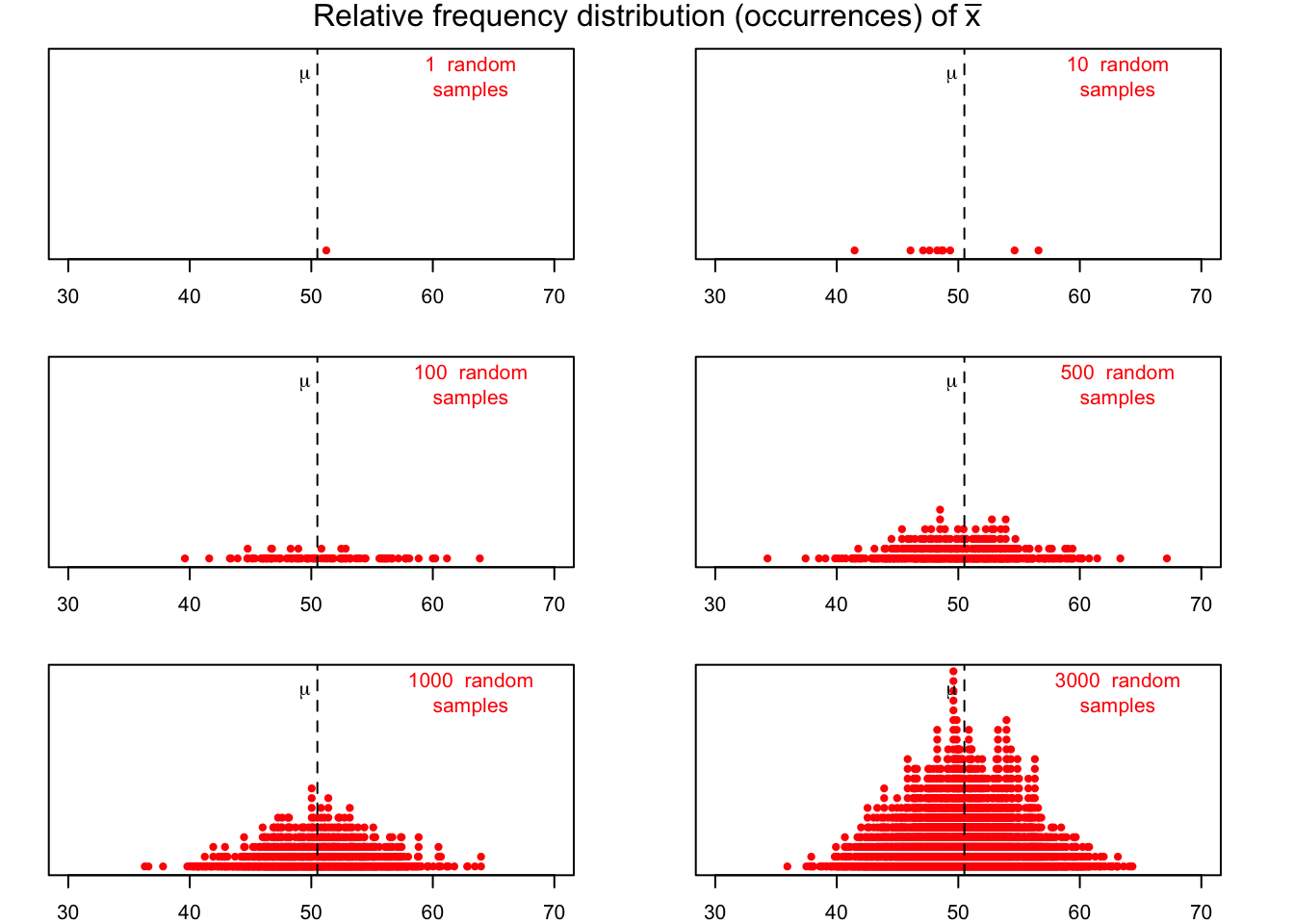Based upon our intuition of randomness in the sampling process, we introduce the Sampling Distribution. The sampling distribution is a distribution of a sample statistic (Lovirc 2010). Often the name of the computed statistic is added as part of the title. For example, if the computed statistic was the sample mean, the sampling distribution would be titled the sampling distribution of the sample mean.

Let us recall the simple example from the previous section, where the population is represented by the first 100 integers $$\{1,2,3,...,100\}$$. If we repeatedly sample from that population and compute each time the sample statistic (e.g. $$\bar x$$ or $$s$$,…), the resulting distribution of sample statistics is a called the sampling distribution of that statistic.

Let us take repeatedly random samples $$(x)$$ without replacement of size $$n = 30$$ from this population. The random sampling might generate sets that look like
$$\{19, 79, 33, 38, 14, 67, 7, 9, 12, 27, 4, 89, 34, 77, 78, 32, 65, 10, 84, 64, 90, 55, 88, 56, 11, 80, 15, 5, 91, 54\}$$
or
$$\{43, 52, 56, 8, 65, 60, 46, 15, 64, 19, 82, 91, 88, 1, 5, 9, 4, 92, 67, 36, 72, 31, 50, 96, 87, 6, 93, 84, 78, 16\}$$, ….
For each sample we calculate a sample statistic. In this example we take the mean, $$\bar x$$, of each sample. However, please note that the sample statistic could be any descriptive statistic, such as the median, the standard deviation, a proportion, among others. Once we obtained the sample means for all samples, we list all their different values and number of their occurrences (frequencies) in order to obtain relative frequencies or empirical probabilities. We turn to R to visualize the relative frequency distribution of repeatedly sampling the given population for 1, 10, 100, 500, 1000, and 3000 times. The sample size is set to $$n=30$$.

pop <- 1:100 # initialize population as all integers between 1 and 100
n <- 30 # sample size

# set ploting parameters
par(mfrow = c(3,2), mar = c(2,2,2,3), xpd = FALSE)

# start experiment
no.samples <- c(1, 10, 100, 500, 1000, 3000) # set number of samples to be drawn

# run experiment 6 times
for (i in 1:length(no.samples)){
# draw either 1, 10, 100, 500, 1000 or 2000 random samples of sample size n=30
my.samples <- rep(NA, no.samples[i]) #initialize empty vector for size i
for (j in 1:no.samples[i]){
# take random samples for j times and calculate the sample mean
my.samples[j] <- mean(sample(pop, n))
}
#plot result (NOTE: Please note that the stripchart function does not scale well.                If you want to experiment with the code you should plot histograms instead)
stripchart(my.samples, method = "stack",
offset = 0.4,
at = .01,
pch = 19,
col = 'red',
xlim = c(30,70))

abline(v = mean(pop), lty = 2)
text(x = mean(pop)*1.25,
y = 1.8,
labels = paste(no.samples[i],' random\nsamples'),
col = 'red')
text(x = mean(pop)*0.98, y = 1.8, labels = expression(mu))
}
mtext(expression(paste("Relative frequency distribution (occurrences) of ", bar(x))), outer=TRUE,  cex=1, line=-1.5)